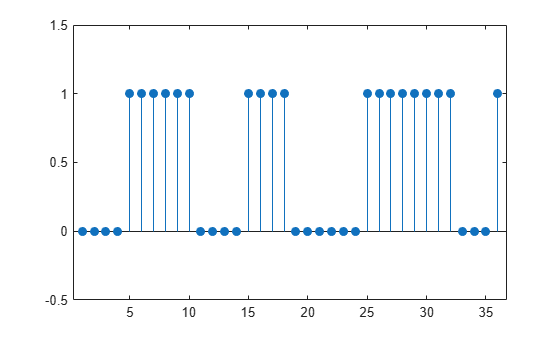Convert matrix of ROI limits to binary mask

## Syntax

``mask = sigroi2binmask(roilims)``
``mask = sigroi2binmask(roilims,len)``

## Description

example

````mask = sigroi2binmask(roilims)` converts `roilims`, a matrix of signal region-of-interest (ROI) limits, to a binary sequence, `mask`, with `true` values indicating samples that belong to regions of interest.```

example

````mask = sigroi2binmask(roilims,len)` specifies the length of the output binary sequence.```

## Examples

collapse all

Consider a two-column matrix of beginning and end samples of four possible regions of interest of a signal. Convert the ROI limits to a logical sequence and display the sequence.

```roilims = [5 10; 15 18; 25 32; 36 38]; mask = sigroi2binmask(roilims); stem(mask,'filled') ylim([0 2]-0.5)```Specify the length of the output sequence as 48. `sigroi2binmask` pads the sequence with false values.

```mask = sigroi2binmask(roilims,48); stem(mask,'filled') ylim([0 2]-0.5)```Specify the length of the output sequence as 36. `sigroi2binmask` ignores samples beyond the specified sequence length.

```mask = sigroi2binmask(roilims,36); stem(mask,'filled') ylim([0 2]-0.5)```## Input Arguments

collapse all

Region-of-interest limits, specified as a two-column matrix of positive integers. The ith row of `roilims` contains nondecreasing indices corresponding to the beginning and end samples of the ith region of interest of a signal.

Example: `[5 8; 12 20; 18 25]` specifies a two-column region-of-interest matrix with three regions.

Data Types: `single` | `double` | `int8` | `int16` | `int32` | `int64` | `uint8` | `uint16` | `uint32` | `uint64`

Output sequence length, specified as an integer scalar. Regions with indices larger than `len` are ignored or truncated. If `len` is greater than `max(roilims(:,2))`, then `sigroi2binmask` pads `mask` with `false` values.

Data Types: `single` | `double` | `int8` | `int16` | `int32` | `int64` | `uint8` | `uint16` | `uint32` | `uint64`

## Output Arguments

collapse all

Binary mask, returned as a logical vector with `true` values indicating samples that belong to a region of interest.

## Version History

Introduced in R2020b